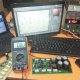Breaking News
Home / Amplifier Diagram / audio amplifier circuit diagram 8 transistors

# audio amplifier circuit diagram 8 transistors

## audio amplifier circuit diagram 8 transistors:

Here in this article, we can learn how to make transistor circuit diagram of 2sc5200 and 2sa1943. We can use 8 transistors in this circuit. 4 pieces of 2sa1943 and 4 pieces of 2sc5200. How many voltages and amperes have to use? We know one transistor can take maximum 1.2 amperes so here in this circuit, we can use 8 transistors. We know Voltage X Ampere = Watts. we use 8 transistors so 1.2X8=9.6. So we can use 9.6 amperes in this circuit. How many Watts can we get from this circuit? We can use 9.6 amperes. If we use 50 Voltage and 8-ampere transformer then we can get Voltage X ampere= Watts, So 50X8=400 Watts. We can use maximum 9.6 this why we use 8 amperes. Please remember that Always use original or good quality one transistor.  transistor audio amplifier circuit diagram

This is the circuit board

## We can Watch the Video of this circuit board:

Center tap transformer for transistor amplifier. transforms is a electrical energy from one circuit to another without any direct electrical connection. We had to use the transformer to everywhere. but nowadays we can use SMPS circuit also. in SMPS also have the center tap. Normally we use Positive and Negative voltage transformer. but center tap has 3 output. Positive, Negative and Ground Voltage. we need this transformer for making the amplifier. normally in a transformer has primary and the secondary wire. for primary we use AC 110v/220 voltage. and we can get output from secondary what’s we need. here secondary has 2 wire output. But in a center tap transformer has 3 wire for the output voltage. For this circuit, we have to use center tap transformer. please share this post if you are like.

transistor circuit diagram of 2sa1943 and 2sc5200

Thanks a lot to be with us. If you want another post then please visit our website.

We have another post for you. like as repairing amplifier,